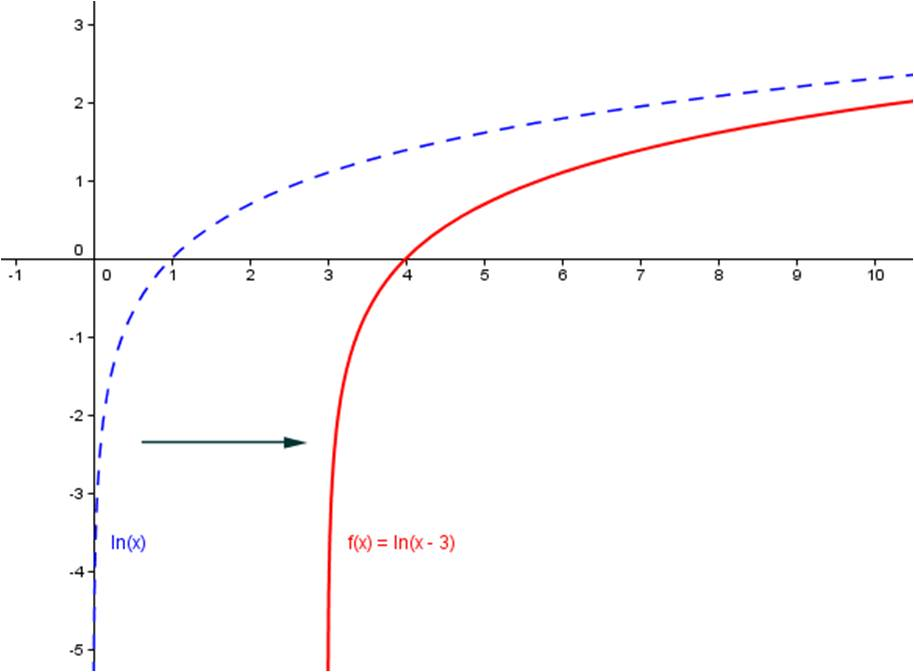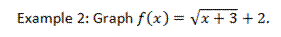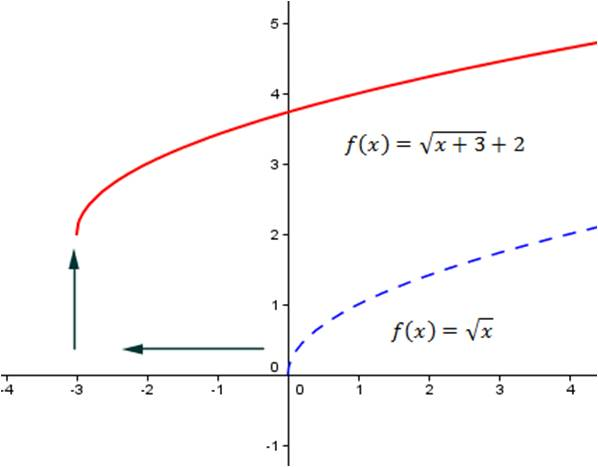## Translating functions

Any function f(x) is translated h units to the right when you replace x with x-h. Any function is translated k units up when you replace y with y-k. This is the basis of the vertex form of parabolas and standard form of the other conic sections, but it will help you graph almost any function you encounter without having to write out a “tree” first. If you know what the “parent” functions look like (and by the time you get to Algebra 2, you should memorize the parent functions), then you can graph translations without too much trouble.

Example 1: Graph

f(x) = ln (x-3).

Here the blue dotted graph is the parent function f(x) = ln (x). The red graph shows the solution, by translating the graph 3 units to the right.In the graphs below, the parent function has been translated 3 units to the left and 2 units up.Algebra 2
Blue Taste Theme created by Jabox